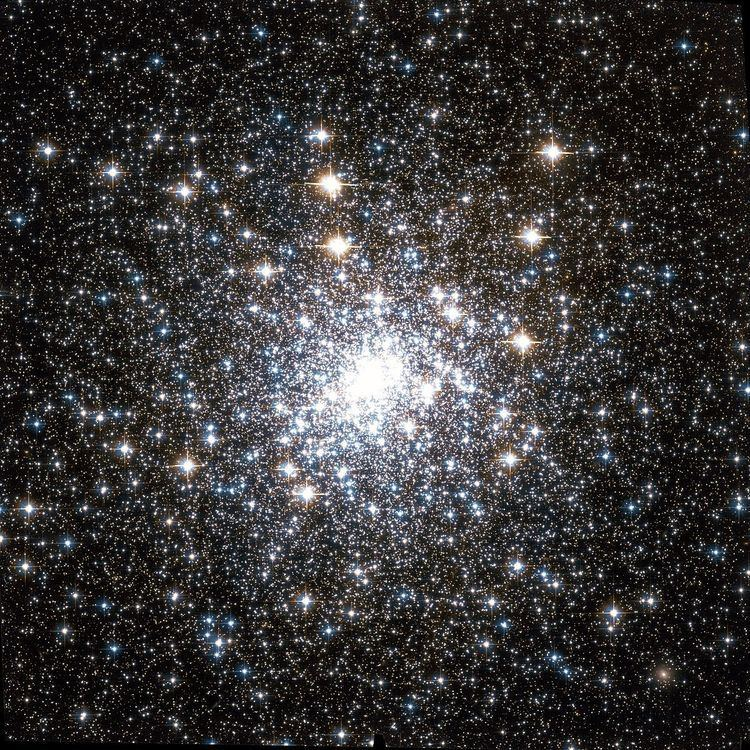# Mass segregation (astronomy)

Updated on
Covid-19In astronomy, dynamical mass segregation is the process by which heavier members of a gravitationally bound system, such as a star cluster or cluster of galaxies, tend to move toward the center, while lighter members tend to move farther away from the center.

## Equipartition of kinetic energy

During a close encounter of two members of the cluster, the members exchange both energy and momentum. Although energy can be exchanged in either direction, there is a statistical tendency for the kinetic energy of the two members to equalize during an encounter; this statistical phenomenon is called equipartition, and is similar to the fact that the expected kinetic energy of the molecules of a gas are all the same at a given temperature.

Since kinetic energy is proportional to mass times the square of the speed, equipartition requires the less massive members of a cluster to be moving faster. The more massive members will thus tend to sink into lower orbits (that is, orbits closer to the center of the cluster), while the less massive members will tend to rise to higher orbits.

The time it takes for the kinetic energies of the cluster members to roughly equalize is called the relaxation time of the cluster. A relaxation time-scale assuming energy is exchanged through two-body interactions was approximated in the textbook by Binney & Tremaine as

t r e l a x = N 8 ln N × t c r o s s   ,

where N is the number of stars in the cluster and t c r o s s is the typical time it takes for a star to cross the cluster. This is on the order of 100 million years for a typical globular cluster with radius 10 parsecs consisting of 100 thousand stars. The most massive stars in a cluster can segregate more rapidly than the less massive stars. This time-scale can be approximated using a toy model developed by Lyman Spitzer of a cluster where stars only have two possible masses ( m 1 and m 2 ). In this case, the more massive stars (mass m 1 ) will segregate in the time

t m 1 = m 2 m 1 × t r e l a x   .

Outward segregation of white dwarfs was observed in the globular cluster 47 Tucanae in a HST study of the region.

## Primordial mass segregation

Primordial mass segregation is non-uniform distribution of masses present at the formation of a cluster. The argument that a star cluster is primordially mass segregated is typically based on a comparison of virilization timescales and the cluster's age. However, several dynamical mechanisms to accelerate virilization compared to two-body interactions have been examined. In star-forming regions, it is often observed that O-type stars are preferentially located in the center of a young cluster.

## Evaporation

After relaxation, the speed of some low mass members can be greater than the escape velocity of the cluster, which results in these members being lost to the cluster. This process is called evaporation. (A similar phenomenon explains the loss of lighter gases from a planet, such as hydrogen and helium from the Earth—after equipartition, some molecules of sufficiently light gases at the top of the atmosphere will exceed the escape velocity of the planet and be lost.)

Through evaporation, most open clusters eventually dissipate, as indicated by the fact that most existing open clusters are quite young. Globular clusters, being more tightly bound, appear to be more durable.

## In the Galaxy

The relaxation time of the Milky Way galaxy is approximately 10 trillion years, on the order of thousand times the age of the galaxy itself. Thus, any observed mass segregation in our galaxy must be almost entirely primordial.

Similar Topics